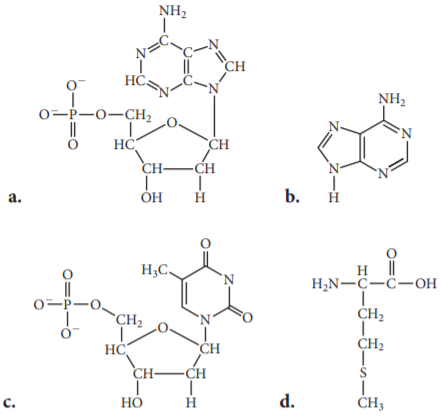×
Get Full Access to Chemistry: A Molecular Approach - 3 Edition - Chapter 21 - Problem 63e
Get Full Access to Chemistry: A Molecular Approach - 3 Edition - Chapter 21 - Problem 63e

×

# Solved: Determine whether or not each structure is aISBN: 9780321809247 1

## Solution for problem 63E Chapter 21

Chemistry: A Molecular Approach | 3rd Edition

• Textbook Solutions
• 2901 Step-by-step solutions solved by professors and subject experts
• Get 24/7 help from StudySoup virtual teaching assistantsChemistry: A Molecular Approach | 3rd Edition

4 5 1 370 Reviews
29
2
Problem 63E

Determine whether or not each structure is a nucleotide. For each nucleotide, identify the base as A, T, C, or G.Step-by-Step Solution:
Step 1 of 3

Solution 63E:Nucleotides are the building blocks of nucleic acid. When a base attaches itself to C-1 of thepentose sugar, a nucleoside is formed. When nucleoside is linked to phosphoric acid at C-5 ofsugar moiety, we get a nucleotide. The general structure of a nucleotide is given below-There are total five bases that may be involved in the formation of a nucleotide.The structure of all the five bases are given below-In the above problem, out of all the options, only (a) and (c) contains a pentose sugar, anitrogenous base and a phosphate group. Hence, option (a) and (c)are nucleotides.Option (b) is adenine(nitrogenous base) whereas option(d) is methionine(amino acid).\na.\nc.In the option (a) base is adenine(A) and in option (c) base is thymine(T).

Step 2 of 3

Step 3 of 3

##### ISBN: 9780321809247

Since the solution to 63E from 21 chapter was answered, more than 453 students have viewed the full step-by-step answer. This textbook survival guide was created for the textbook: Chemistry: A Molecular Approach, edition: 3. Chemistry: A Molecular Approach was written by and is associated to the ISBN: 9780321809247. The full step-by-step solution to problem: 63E from chapter: 21 was answered by , our top Chemistry solution expert on 02/22/17, 04:35PM. This full solution covers the following key subjects: nucleotide, base, identify, determine, structure. This expansive textbook survival guide covers 82 chapters, and 9454 solutions. The answer to “?Determine whether or not each structure is a nucleotide. For each nucleotide, identify the base as A, T, C, or G.” is broken down into a number of easy to follow steps, and 21 words.

## Discover and learn what students are asking

Calculus: Early Transcendental Functions : Functions of Several Variables
?In Exercises 15 - 22, find all first partial derivatives. $$f(x, y)=y^{3} e^{4 x}$$

Calculus: Early Transcendental Functions : Parametric Equations and Calculus
?In Exercises 1-4, find $$d y / d x$$. $$x=\sqrt{t}, y=4-t$$

Statistics: Informed Decisions Using Data : Testing the Significance of the Least-Squares Regression Model
?True or False: In a least-squares regression, the response variable is normally distributed with mean my!x and standard deviation s.

Unlock Textbook Solution